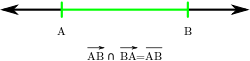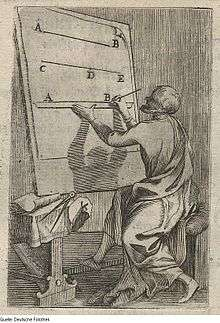# Line segment

Not to be confused with Curved line segment.The geometric definition of a closed line segment: the intersection of all points at or to the right of A with all points at or to the left of Bhistorical image – create a line segment (1699)

In geometry, a line segment is a part of a line that is bounded by two distinct end points, and contains every point on the line between its endpoints. A closed line segment includes both endpoints, while an open line segment excludes both endpoints; a half-open line segment includes exactly one of the endpoints.

Examples of line segments include the sides of a triangle or square. More generally, when both of the segment's end points are vertices of a polygon or polyhedron, the line segment is either an edge (of that polygon or polyhedron) if they are adjacent vertices, or otherwise a diagonal. When the end points both lie on a curve such as a circle, a line segment is called a chord (of that curve).

## In real or complex vector spaces

If V is a vector space overor, and L is a subset of V, then L is a line segment if L can be parameterized asfor some vectors, in which case the vectors u and u + v are called the end points of L.

Sometimes one needs to distinguish between "open" and "closed" line segments. Then one defines a closed line segment as above, and an open line segment as a subset L that can be parametrized asfor some vectors.

Equivalently, a line segment is the convex hull of two points. Thus, the line segment can be expressed as a convex combination of the segment's two end points.

In geometry, it is sometimes defined that a point B is between two other points A and C, if the distance AB added to the distance BC is equal to the distance AC. Thus inthe line segment with endpoints A = (ax, ay) and C = (cx, cy) is the following collection of points:.

## Properties

• A line segment is a connected, non-empty set.
• If V is a topological vector space, then a closed line segment is a closed set in V. However, an open line segment is an open set in V if and only if V is one-dimensional.
• More generally than above, the concept of a line segment can be defined in an ordered geometry.
• A pair of line segments can be any one of the following: intersecting, parallel, skew, or none of these. The last possibility is a way that line segments differ from lines: if two nonparallel lines are in the same Euclidean plane they must cross each other, but that need not be true of segments.

## In proofs

In an axiomatic treatment of geometry, the notion of betweenness is either assumed to satisfy a certain number of axioms, or else be defined in terms of an isometry of a line (used as a coordinate system).

Segments play an important role in other theories. For example, a set is convex if the segment that joins any two points of the set is contained in the set. This is important because it transforms some of the analysis of convex sets to the analysis of a line segment. The Segment Addition Postulate can be used to add congruent segment or segments with equal lengths and consequently substitute other segments into another statement to make segments congruent.

## As a degenerate ellipse

A line segment can be viewed as a degenerate case of an ellipse in which the semiminor axis goes to zero, the foci go to the endpoints, and the eccentricity goes to one. As a degenerate orbit this is a radial elliptic trajectory.

## In other geometric shapes

In addition to appearing as the edges and diagonals of polygons and polyhedra, line segments appear in numerous other locations relative to other geometric shapes.

### Triangles

Some very frequently considered segments in a triangle include the three altitudes (each perpendicularly connecting a side or its extension to the opposite vertex), the three medians (each connecting a side's midpoint to the opposite vertex), the perpendicular bisectors of the sides (perpendicularly connecting the midpoint of a side to one of the other sides), and the internal angle bisectors (each connecting a vertex to the opposite side). In each case there are various equalities relating these segment lengths to others (discussed in the articles on the various types of segment) as well as various inequalities.

Other segments of interest in a triangle include those connecting various triangle centers to each other, most notably the incenter, the circumcenter, the nine-point center, the centroid, and the orthocenter.

In addition to the sides and diagonals of a quadrilateral, some important segments are the two bimedians (connecting the midpoints of opposite sides) and the four maltitudes (each perpendicularly connecting one side to the midpoint of the opposite side).

### Circles and ellipses

Any straight line segment connecting two points on a circle or ellipse is called a chord. Any chord in a circle which has no longer chord is called a diameter, and any segment connecting the circle's center (the midpoint of a diameter) to a point on the circle is called a radius.

In an ellipse, the longest chord is called the major axis, and a segment from the midpoint of the major axis (the ellipse's center) to either endpoint of the major axis is called a semi-major axis. Similarly, the shortest chord of an ellipse is called the minor axis, and the segment from its midpoint (the ellipse's center) to either of its endpoints is called a semi-minor axis. The chords of an ellipse which are perpendicular to the major axis and pass through one of its foci are called the latera recta of the ellipse. The interfocal segment connects the two foci.

## Directed line segment

When a line segment is given an orientation (direction) it suggests a translation or perhaps a force tending to make a translation. The magnitude and direction are indicative of a potential change. This suggestion has been absorbed into mathematical physics through the concept of a Euclidean vector. The collection of all directed line segments is usually reduced by making "equivalent" any pair having the same length and orientation. This application of an equivalence relation dates from Giusto Bellavitis’s introduction of the concept of equipollence of directed line segments in 1835.

## Generalizations

Analogous to straight line segments above, one can define arcs as segments of a curve.Wikimedia Commons has media related to Line segment.Look up line segment in Wiktionary, the free dictionary.